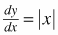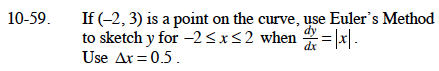### Home > CALC > Chapter 10 > Lesson 10.1.6 > Problem10-59

10-59.

If (−2, 3) is a point on the curve, use Euler's Method to sketch y for −2 ≤ x ≤ 2 when. Use ∆x = 0.5. Homework Help ✎If x = –2, then dy = 1.
Therefore the next point is (–2 + 0.5, 3 + 1) or (–1.5, 4).